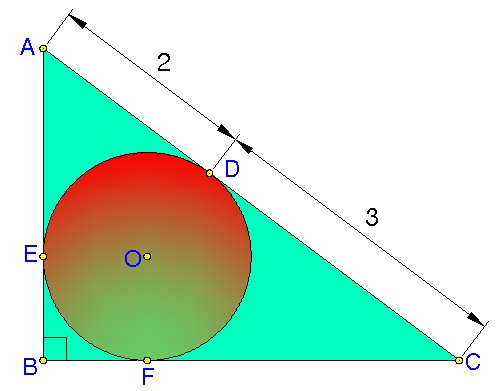# A geometry problem by A Former Brilliant MemberCircle $O$ is inscribed in $\triangle ABC,$ as shown in the figure above. The points of tangency are at $D,E,$ and $F$. Given that $AD=2$ and $DC=3$, find the area of $\triangle ABC$.

×